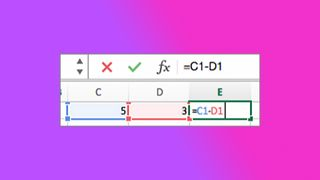# How to subtract in Excel — make Microsoft do the math for youExcel can be a confusing landscape of chaos if you don't know what you're doing, so something simple like subtracting ends up being a nightmare.

Well, we're adding a few rainbows and unicorns to those dreams and we're going to teach you the ways of Excel, that is how to subtract in Excel. We've already written about how to remove duplicate entries in Excel (if you're interested in that instead).

Otherwise, here's how to subtract in Excel.

## How to subtract in Excel

Subtract two or more numbers in a cell

Step 1) Select a blank cell and type an equal size [=] to start.

Step 2) After the equal sign, type out the desired numbers and separate them with a minus sign (-). Example: 43-3-23-12

Step 3) Press the Return key. With the example above, you should get 5.

Subtract numbers using cell references

Step 1) Type a number in the desired cells. For example, cells A1 and B1 with numbers 7 and 4, respectively.

Step 2) Select the cell you wish to have your total (like C1) and type the equal sign [=].

Step 3) After the equal sign, type the cells you wish to subtract. If you're following our example, it would be A1-B1.

Step 4) Now just press the Return key. Our result should be 3.

It's as simple as that. There's not too much to it, so don't go pulling your hair out over Excel. There are plenty of tips and tricks out there, many of which can be found right here.

CategoryBack to Ultrabook Laptops
BrandProcessorRAMStorage SizeScreen SizeColourConditionPriceAny Price